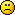# What are the uses of limits and differentiation

1. What are the uses of limits and differentiation

2. way too many to list all in one place as both are fairly foundational to a lot of branches of mathematics.

The most immediate thing I can think of is optimization. Derivatives are often used to find the maximum or minimum value of a function.

3. When I think of differentiation I usually think of a rate of change (although this is only for equations differentiated with respect to t = time).  For example, in classical mechanics, the acceleration is normally expressed as the derivative (with respect to t) of the velocity equation.  The inverse operation is known as integration.

The derivative of y=f(x) with respect to x will allow you to determine how 'steep' the curve f(x) for each value of x. You may use the equation for the derivative to determine for what values x gives a 0 derivative, this indicates the points where the curve is 'flat'.  These are often the most 'interesting' points i.e. mimimum or maximum values.  (Note a derivative of 0 does not necessarily indicate a maximum or minimum).

The limit of a function lim x->a (f(x)) tells you what will happen to f(x) as x approaches a.  This is often useful where f(a) itself may not be defined, or where lim x->a f(x) does not equal f(a).

For example, if we wish to find out what happens as x approaches infinity for y1 - 1/x), we cannot simply substitute x with infinity as it is not a real number, we are instead going to see what happens as x gets very large but remains real.

It is clear that 1/x gets very small as x gets very large, and with some analysis we can show that no matter how small we would like 1/x to be, there is some x>0 which will make 1/x at least as small as desired.  This means the limit as x approaches infinity of y1-1/x) will equal 1, as lim x-> infinity of 1/x is 0.

working